Tutorial Contents Tutorial Five: Predicate Calculus: Tableaux - The \$xj rule - Bad Mistakes and Technical Mistakes - Ticking - Finished Tableaux - Sequents - Soundness and Completeness - Proof of a syntactic sequent: example More Tutorials One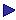TwoThreeFourSixSeven# 5: Predicate Calculus: Tableaux

### Predicate Tableaux

There are four new rules to deal with the quantifiers: one each for "xj (and, of course, for "yj, etc), and for ¬"xj; and one each for \$xj and ¬\$xj. In addition to these we retain the rules for propositional tableaux, except that we dispense with ticking. (See the paragraph on ticking below.)

#### The "xj rule

The rule is: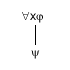where there is a designator D which has already occurred in the branch to which y is added, and y is the result of replacing each free occurrence of "x" in j by an occurrence of D. (i.e. the rule is that we can add "y" to any open branch in which ""xj" occurs where this condition is satisfied.)

Note particularly:

1. "D" must already occur in the branch;

2. The rule applies only to formulae which begin with """.

The rationale of this rule is that, if something is true of everything, it is true in particular of whatever D stands for  assuming that it stands for something (see below: example (vi)).

Example:  a proof that {Fa, "x[Fx®Gx], "x¬Gx} is inconsistent: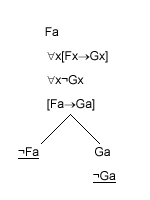The tableau closes; so the original set is inconsistent.

The following are correct applications of the rule:

 (i) Ha "zFz   Fa

(The rule applies to "y, "z , etc as well as "x)

(ii)

 "y[\$x[Gx®Ray]ÙFy] [\$x[Gx®Raa]ÙFa]

("a" occurs in the branch to which "[\$x[Gx®Raa]ÙFa]" is added; it doesn't need to

occur higher than the line to which the rule is applied.)

The following are incorrect attempts to apply the rule; the incorrect applications are starred.

 (iii) Fa ¬"xFx *¬Fa

Wrong, because the rule applies only to formulae beginning with """. If this application were correct, we would be able to "prove" the following inconsistent: {Buttercup is brown, not everything is brown}.

 (iv) ¬¬"xFx ¬Fa    *¬¬Fa

Again the mistake consists in applying the rule to a formula which does not begin with """. The following would have been correct:

 ¬¬"xFx ¬Fa "xFx Fa

 (v)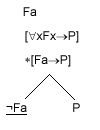Again the mistake consists in applying the rule to a formula which does not begin with """. The following would have been correct: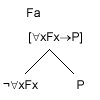But now the left branch does not close.

 (vi) "xFx "x¬Fx *Fa ¬Fa

The mistake is that the "a" which is introduced in "Fa" has not occurred already in the branch. The tableau is a faulty attempt to prove that {"xFx, "x¬Fx} is inconsistent. But it is not: in the empty domain both ""xFx" and ""x¬Fx" will be true.

The point of the requirement that the designator should already have occurred in the branch is to block such a faulty proof. One can look at it like this: the use of "a" is permitted only if "a" names something; but since "a" does not already occur in the branch, "a" may not name anything at all.

#### Note: disallowing the empty domain

Hodges's tableau system is designed to allow for the possibility that we may be dealing with an empty domain. Most systems, on the other hand, allow us to assume that we are not dealing with an empty domain. But if we are not {"xFx, "x¬Fx} will after all be inconsistent, and there is no need to require that the designator which is introduced by the "xj rule already occurs in the branch. After all, if we are allowed to assume that the domain is not empty, we may safely assume that there is something for the designator to name.

If one wishes to disallow the empty domain, then, one way of doing so is to alter the "xj rule. An alternative is to introduce a new rule which applies to cases where there is no designator in the branch in which "xj occurs. Hodges suggests adopting the following rule (p. 192):

#### Rule VIIwhere no designators have occurred in the branch to which y is added, D is a proper name and y is the result of replacing each free occurrence of "x" in j by an occurrence of D.

You should note, however, that Hodges himself does NOT adopt this rule